Next: The prior of the Up: The probabilistic model Previous: The probabilistic model   Contents

### The likelihood

Let us assume that the noise terms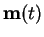and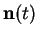in Equation (5.18) are all independent at different time instants and Gaussian with zero mean. The different components of the vector are, however, allowed to have different variances as governed by their hyperparameters. Following the developments of Section 3.1.1, the model implies a likelihood for the data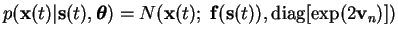(5.21)

where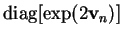denotes a diagonal matrix with the elements of the vector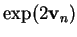on the diagonal. The vector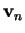is a hyperparameter that defines the variances of different components of the noise.

As the values of the noise at different time instants are independent, the full data likelihood can be written as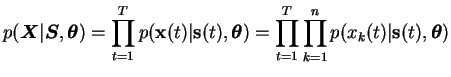(5.22)

where the individual factors are of the form(5.23)Next: The prior of the Up: The probabilistic model Previous: The probabilistic model   Contents
Antti Honkela 2001-05-30